• Online Training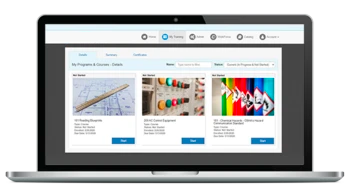• Instructor-Led Training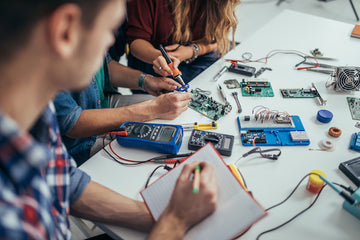• Performance• Compliance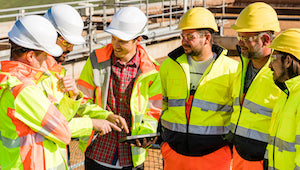• TECHNOLOGY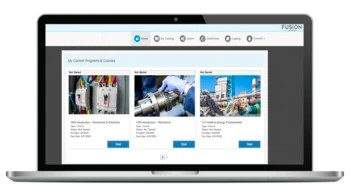• Industries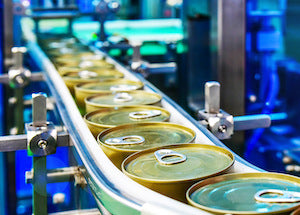• Resources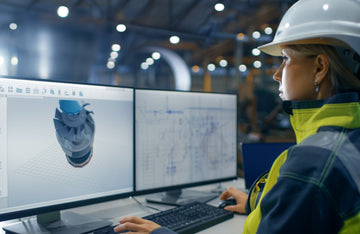• Contact
• ## Industrial Fundamentals Online Training Courses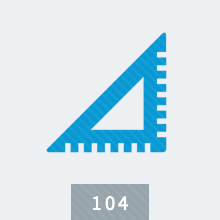# Making Measurements

"Making Measurements" covers units of measurement used in commercial and industrial applications. Examines all aspects of basic measurement concepts and procedures, including accuracy and tolerance. This online course discusses techniques and devices for comparison measurements (dial indicators and gauge blocks). Shows common methods for measuring volume, motion, force, temperature, fluid flow, and electricity. Explains how to use scales and rules, combination calipers, and micrometers. This course has no prerequisites. Making Measurements is available in online technical training and course manual formats.

Review a full training course list for Millwright

#### Lesson 1 - Units of Measurement

##### Topics:

Length; Area; Volume; Angles; Time; Speed and velocity; Mass and weight; Force; Work and power; Pressure; Temperature; Electricity

##### Learning Objectives:
– Identify various units of measurement.
– State the definition of the joule, the coulomb, and the horsepower
– Explain how to calculate pressure.
– Explain the difference between mass and weight.
– Demonstrate how to measure the volume of an object.
– Explain the difference between the Celsius scale and the Fahrenheit scale.

#### Lesson 2 - Metric Measurement

##### Topics:

Length, area, volume; Mass; Frequency; Speed and velocity; Acceleration; Force and weight; Work, energy, and power; Temperature; Current; Light

##### Learning Objectives:
– List the seven base units in the SI (metric) system.
– Name three derived units.
– Define work and power in SI units.
– Explain what power is and how it is measured.
– Name two metric measuring instruments and their U.S. Standard equivalents.

#### Lesson 3 - Linear Measurement

##### Topics:

Units; Measurement error; Tolerances; Scales and rules; Scribers and dividers; Bevel gauge; Calipers; Combination square; Using a micrometer

##### Learning Objectives:
– List five units used for making linear measurements.
– Demonstrate how to use a micrometer.
– Explain what each head of a combination square is used for.
– State the definition of parallax error.
– Define the different types of tolerance.

#### Lesson 4 - Comparison and Surface Measurement

##### Topics:

Gauge blocks; Measuring screw threads, radius, surface texture; Hardness testing; Testing surface coatings; Detecting defects

##### Learning Objectives:
– Explain the difference between a continuous dial and a balanced dial on a dial indicator.
– State the definition of pitch on a screw.
– Name two hardness tests.
– Explain why nondestructive testing is preferable to destructive testing on surface coatings.

#### Lesson 5 - Measuring Bulk Materials

##### Topics:

Storing and handling bulk solids; Conveyors; Measuring area, volume, weight, mass, density; Measuring lumber

##### Learning Objectives:
– Explain why weight-density and the angle of repose are important to workers who handle and store loose bulk material.
– Name the two types of conveyors and list three specific examples of each type.
– Name the three basic measurements of bulk materials.
– Demonstrate how to find the radius of a circle, given its area, and how to find the area of a circle, given its circumference.
– Demonstrate how to convert a typical order of lumber into board feet.

#### Lesson 6 - Measuring Motion

##### Topics:

Relative motion; Displacement; Velocity; Acceleration; Average and instantaneous values; Motion on a curved path; Graphs of motion

##### Learning Objectives:
– Name the three measurements of motion.
– State the definition of speed.
– Explain the difference between average and instantaneous velocity.
– Demonstrate how to interpret a graph of motion.
– Explain of the velocity of an object is shown on a graph of motion.

#### Lesson 7 - Measuring Forces

##### Topics:

How forces act; Combining forces; Force and torque measurement; Analyzing forces

##### Learning Objectives:
– Name both the metric and the U.S. Standard units of measurement for force, mass, and acceleration.
– State the definition of force.
– Demonstrate how to calculate torque.
– State an advantage of using a balance instead of a scale.
– Demonstrate how to draw a force diagram.

#### Lesson 8 - Measuring Temperature

##### Topics:

Temperature and heat; Temperature-sensing materials; Thermometers; Pyrometers; Response time and accuracy

##### Learning Objectives:
– Explain the difference between heat and temperature.
– Name four different scales for measuring temperature.
– Explain the use of heat-sensitive pellets, crayons, and paints.
– Explain how Bourdon tubes work.
– Explain how a pyrometer works.

#### Lesson 9 - Measuring Fluids

##### Topics:

States of matter; Measuring liquid level, viscosity, flow rate, volume of flow, specific gravity, pressure, humidity, density

##### Learning Objectives:
– State the definition of a fluid.
– Describe how liquids differ from gases.
– List the instruments used to measure the level of water.
– Name two instruments that measure the flow of fluids, and explain how they work.

#### Lesson 10 - Measuring Electricity

##### Topics:

Electricity; Electric circuits; Electrical units; Measuring current, potential difference, resistance, power; AC and DC measurements

##### Learning Objectives:
– List the parts of an atom.
– Define potential difference.
– Identify a wattmeter.
– Describe the difference between alternating current and direct current.
– Describe the difference between an ohmmeter and an ammeter. Back to Industrial Fundamentals Online Training Courses Courses

#### Select Format

• Online Course & Test \$85.00